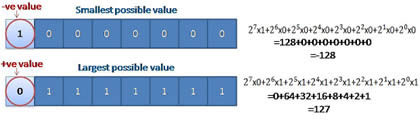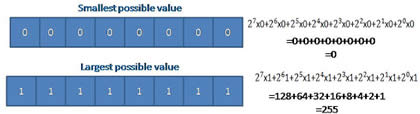# Difference between Signed Char and Unsigned Char

##### Key Difference: Signed char and unsigned char are two data types used in C programming. Both unsigned and signed char are used to store characters and consist of an area of 8 bits. Unsigned characters have values between 0 and 255, whereas signed characters have values from –128 to 127 (on a machine with 8 bit bytes and two's complement arithmetic).Char is a data type which is used in C programming for storing characters like letters and punctuation marks. However, it still remains to be an integer type. This is due to the reason that char type technically stores integers and not characters. It makes use of a numerical code which represents characters by using integers. For example – ASCII code which is one of the most commonly used codes for carrying out such interpretations.

C90 enabled C to use keywords signed and unsigned with char. It is important to note that they are only used when small integer values are to be handled. Signed char and unsigned char are both of 8 bit bytes on a 16 bit machine. They primarily differ in the range represent by them. The range of unsigned char is 0 to 255, whereas the size of char or signed char is -128 to 127.The transformation of char into int values is done automatically by C. However, it is still dependent on the machine which decides that the result would be negative or not. The upper case A is equivalent to integer value of 65.

The terms unsigned and signed used with char means that if the content of these eight bits is interpreted into an integer then these terms can make some difference. Character data type is usually of type unsigned by default. However, In C++ and ANSI C mode, there is an option to explicitly declare them as signed or unsigned char. In unsigned char 8 bits are used as data bits, whereas in memory representation of signed char 1 bit (most significant bit) is used for signed bit and 7 bits are used as data bits. If the signed bit is 0 it means that number is positive. If signed bit is 1 then number is negative.

Comparison between Signed Char and Unsigned Char:

 Signed Char Unsigned Char Range -128 to +127 0 to 255 Typical bit width 1 byte 1 byte Example (x=10000010) Signed char x = -2 Unsigned char = 82 Representation 1 bit = signed bit 7 bit = data bits 8 bit = data bits Declaration Signed char b Unsigned char a

Images Courtesy: lekhrajkullu.blogspot.in